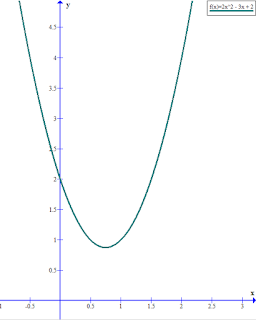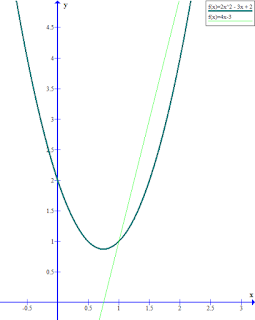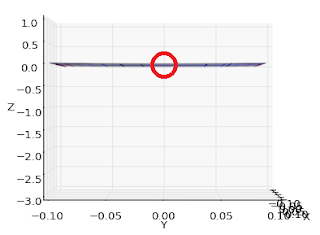## Saturday, January 23, 2016

Algebraic method: 1 variable

Say you have an equation like
y = 2x^2 - 3x + 2
that you needed to find the minimum of:The quadratic equation is minimum (the turning point at the bottom) somewhere between x = 0.5 and x = 1. How can we find where exactly it is minimum? The standard solution is by algebraically finding where its gradient is 0. The gradient is 0 only at a turning point, which is where the minimum is. You can find the gradient at any point by taking the equation's derivative. The derivative with respect to "x" of y = 2x^2 - 3x + 2 is
dy/dx = 4x - 3The derivative equation is 0 exactly at the same point where the first equation has a minimum turning point. So we algebraically find at which "x" "4x - 3" is 0:
0 = 4x - 3
x = 3/4 = 0.75

Therefore "x" has to be 0.75.

Algebraic method: 2 variables

What if we have 2 variables instead of just "x"? This is what the equation
z = 2x^2 + 2y^2 + 2xy - 6x
looks like:It clearly has a minimum point somewhere close to (x,y) = (0,0). The way to find the minimum is to take it's partial derivative with respect to "x" and with respect to "y". A partial derivative is when you consider only one of the variables as a variable whilst considering the other variables as constant. This allows you to find how the gradient of the curve changes with respect to one variable. Here is the partial derivative with respect to "x":
∂z/∂x = 4x + 2y - 6
and here is the partial derivative with respect to "y":
∂z/∂y = 4y + 2x

The idea is now to find where the gradient with respect to both variables is 0. The minimum has a gradient of zero from all directions ("x" and "y") so we find where this is so:
0 = 4x + 2y - 6 [A]
0 = 4y + 2x [B]

From [B]
x = -2y [C]

Substituting [C] in [A]
0 = 4(-2y) + 2y - 6
y = -1

Therefore from [C]
x = 2


Therefore both derivatives are equal to 0 when (x,y) = (2,-1) which means that that is where the equation is minimum.

Notice how finding the the minimum of a 2 variable equation required the use of solving simultaneous equations. Imagine having a 100 variables and solving 100 simultaneous equations. Plus there is no guarantee that the equations are solvable algebraically. So sometimes we use approximate methods to find the minimum. In this case we can use a method called Gradient Descent. You start by finding the partial derivatives with respect to each variable, then you pick a point on the equation at random and follow the gradient downwards. Let's see an example with the previous equation.

∂z/∂x = 4x + 2y - 6
∂z/∂y = 4y + 2x


Let's pick (x,y) = (0,0) as a point.

∂z/∂x @ (0,0) = 4(0) + 2(0) - 6 = -6
∂z/∂y @ (0,0) = 4(0) + 2(0) = 0


The gradients suggest that at (0,0) the curve is flat at the "y" axis and sliding downwards in the positive direction of the "x" axis. Here is what that means.

If we fix "y" to be 0, "z" would vary with respect to "x" like this:Notice how at "x" = 0 the slope steeps down towards the positive direction, which means that "x" = 0 is too small and needs to be bigger for us to find the minimum. The gradient at "x" = 0 is -6, which is negative therefore the minimum is at a greater "x" than 0.

If we fix "x" to be 0, "z" would vary with respect to "y" like this:Notice how at "y" = 0 the slope is flat, which means that "y" is not important to find the minimum at this point and can be left as is. The gradient at "y" = 0 is 0, therefore we can leave "y" as is.

In that case, we stay where we are in the "y" axis direction and increase the "x". By how much should we increase? Is the point (1,0) closer to the minimum than (0,0)? Is it too small an increase? Would (10,0) be a better new point? Or is it too big and should be (0.1,0) instead? How can we get a new point which is closer to the minimum than (0,0) is?

As we approach the minimum, the gradients will start flattening out in both the "x" and "y" directions and start getting closer to 0. This means that we can, as a rule of thumb, increase or decrease the "x" and "y" in the point in proportion to the gradient. The larger the gradient, the steeper the slope, so the more promising moving in that direction would be to find the minimum. On the other hand, the smaller the gradient, the flatter the slope, so moving in that direction would not be very profitable as we're already close to the minimum there and might overshoot. If the gradient is zero, then we are right where we want to be and shouldn't move in that axis at all. So the greater the gradient, the greater the distance we should move.

It's important to understand that the step size should be in proportion to the gradient, not exactly the gradient. We should move at a fraction of the gradient since the gradient will usually be really big compared to the distance required to move and will probably overshoot all the time if used as is. Let's use 0.1 of the gradient.

new x = 0 - 0.1(-6) = 0.6
new y = 0 - 0.1(0) = 0


Notice that we subtracted the gradient so that if the gradient is negative we move towards the positive direction whilst if the gradient is positive we move towards the negative direction since that is how the slope will be oriented.

We check the gradients again to see if we're there yet:

∂z/∂x @ (0.6,0) = 4(0.6) + 2(0) - 6 = -3.6
∂z/∂y @ (0.6,0) = 4(0) + 2(0.6) = 1.2


At our new point we're at a slope sliding downwards towards the positive direction in the "x" and towards the negative direction in the "y" direction. Here are the graphs to confirm.

If we fix "y" to be 0, "z" would vary with respect to "x" like this:Notice how at "x" = 0.6 the slope steeps down towards the positive direction. The gradient at "x" = 0 is -3.6.

If we fix "x" to be 0.6, "z" would vary with respect to "y" like this:Notice how at "y" = 0 the slope is steeps down towards the negative directions. The gradient at "y" = 0 is 1.2.

So we need to make "x" bigger and "y" smaller.

new x = 0.6 - 0.1(-3.6) = 0.96
new y = 0 - 0.1(1.2) = -0.12


And we keep on reiterating until the gradient is sufficiently close to 0 in both the "x" and "y" directions or until the change in both "x" and "y" is small enough.

Here's a list of the first 20 points we'll get:
(0, 0)
(0.6, 0.0)
(0.96, -0.12)
(1.2, -0.26)
(1.37, -0.4)
(1.5, -0.51)
(1.6, -0.61)
(1.68, -0.69)
(1.75, -0.75)
(1.8, -0.8)
(1.84, -0.84)
(1.87, -0.87)
(1.9, -0.9)
(1.92, -0.92)
(1.93, -0.93)
(1.95, -0.95)
(1.96, -0.96)
(1.97, -0.97)
(1.97, -0.97)
(1.98, -0.98)

The point gets closer and closer to the actual minimum which is (2,-1). Here are the illustrated points:See how as the point approaches the minimum it starts moving less and less?

This is called the Gradient Descent algorithm. We can fine-tune its performance by varying the starting point and the step size fraction by which we're multiplying the gradient.

Here's a Python 3 program that performs Gradient Descent given a list of gradient functions (for each variable):
def grad_desc(gradients, initial_values, step_size, threshold):
old_values = initial_values
while True:

grad_desc([ lambda x,y:4*x+2*y-6, lambda x,y:4*y+2*x ], [0, 0], 0.1, 0.001)